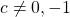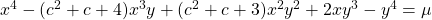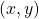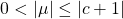# A parametric family of quartic Thue inequalities

Salah Eddine Rihane, Mohand Ouamar Hernane and Alain Togbé
Notes on Number Theory and Discrete Mathematics
Print ISSN 1310–5132, Online ISSN 2367–8275
Volume 27, 2021, Number 4, Pages 1—14
DOI: 10.7546/nntdm.2021.27.4.1-14

## Details

### Authors and affiliations

Salah Eddine RihaneDepartment of Mathematics and Computer Science
Abdelhafid Boussouf University
Mila 43000, Algeria

Mohand Ouamar HernaneUniversité des Sciences et de la Technologie Houari-Boumedièene (USTHB),
Faculté de Mathématiques, Laboratoire d’Algèbre et Théorie des Nombres
BP 32, 16111 Bab-Ezzouar, Alger, Algerie

Alain TogbéDepartment of Mathematics, Statistics
Purdue University Northwest
1401 S, U.S. 421, Westville IN 46391, United States

### Abstract

Letbe an integer. In this paper, we use the method of Tzanakis to transform the quartic Thue equationinto systems of Pell equations. Then, we determine all primitive solutionswith.

### Keywords

• Parametric Thue equations
• Method of Tzanakis
• Continued fraction
• Linear form in logarithms

• 11D59
• 11D09
• 11D75
• 11D25
• 11A55
• 11J86

### References

1. Dujella, A. (2004). Continued fractions and RSA with small secret exponent. Tatra Mountains Mathematical Publications, 29, 101–112.
2. Dujella, A., & Ibrahimpašic, B. (2008). On Worley’s theorem in Diophantine approximations. Annales Mathematicae et Informaticae, 35, 61–73.
3. Dujella, A., Ibrahimpašic, B., & Jadrijević, B. (2011). Solving a family of quartic Thue inequalities using continued fractions. Rocky Mountain Journal of Mathematics, 41(4), 1173–1182.
4. Dujella, A., & Jadrijević, B. (2002). A parametric family of quartic Thue equations. Acta Arithmetica, 101(2), 159–170.
5. Dujella, A., & Jadrijević, B. (2004). A family of quartic Thue inequalities. Acta Arithmetica, 111(1), 61–76.
6. Dujella, A., & Pethő, A. (1998). A generalization of a theorem of Baker and Davenport, The Quarterly Journal of Mathematics Oxford Series (2), 49(3), 291–306.
7. He, B., Jadrijević, B. & Togbé, A. (2009) Solutions of a class of quartic Thue inequalities. Glasnik Matematicki Series III, 44, 309–321.
8. He, B., Kihel, O., & Togbé, A. (2011). Solutions of a class of quartic Thue inequalities. Computers & Mathematics with Applications, 61, 2914–2923.
9. Jadrijević, B. (2011). A parametric family of quartic Thue inequalities. Bulletin of the Malaysian Mathematical Sciences Society, 34(2), 215–230.
10. Ibrahimpašic, B. (2013). Explicit version of Worley’s theorem in Diophantine approximations. Bulletin of International Mathematical Virtual Institute, 3, 59–68.
11. Jadrijević, B. (2005). A system of Pellian equations and related two-parametric family of quartic Thue equations. Rocky Mountain Journal of Mathematics, 35(2), 547–571.
12. Jadrijević, B. (2005). On two-parametric family of quartic Thue equations.  Journal de Theorie des Nombres de Bordeaux, 17, 161–167.
13. Keskin, R., & Duman, M. G. (2019). Positive Integer Solutions of Some Pell Equations. Palestine Journal of Mathematics, 8(2), 213–226.
14. Laurent, M. (2008). Linear forms in two logarithms and interpolation determinants II. Acta Arithmetica, 133(4), 325–348.
15. Leprevost, F., Pohst, M., & Schöpp, A. (2007). Units in some parametric families of quartic fields. Acta Arithmetica, 127(3), 205–216.
16. Matveev, E. M. (2000). An explicit lower bound for a homogeneous rational linear form in logarithms of algebraic numbers II. Izvestiya: Mathematics, 64(6), 1217–1269.
17. Tzanakis, N. (1993). Explicit solution of a class of quartic Thue Equations. Acta Arithmetica, 64(3), 271–283.
18. Worley, R. T. (1981). Estimating |αp/q|. Journal of the Australian Mathematical Society, 31(2), 202–206.
19. Ziegler, V. (2006). On certain family of quartic equations with three parameters. Glasnik Matematicki Series III, 41(1), 9–30.

## Cite this paper

Rihane, S. E., Hernane, M. O., & Togbé, A. (2021). A parametric family of quartic Thue inequalities. Notes on Number Theory and Discrete Mathematics, 27(4), 1-14, doi: 10.7546/nntdm.2021.27.4.1-14.